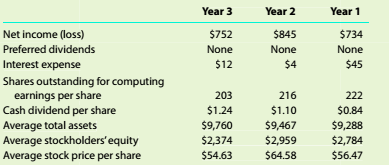Chapter 9, Problem 9.4.1C

Chapter
Section
Textbook Problem

Profitability and stockholder ratios Harley-Davidson, Inc. (HOG), is a leading motorcycle manufacturer in the United States. The company manufactures and sells a number of different types of motorcycles, a complete line of motorcycle parts, and brand-related accessories, clothing, and collectibles. The following information is available for three recent years (in millions except per-share amounts):Calculate the following ratios for each year. Round to one decimal place except dollar amounts, which should he rounded to the nearest cent. a.Return on total assets b.Return on stockholders' equity c.Earnings per share d.Dividend yield e.Price-earninus ratio using the average price oer share of stock.

To determine

Introduction:

Accounting ratios are used to evaluate the financial performance of the business organisation

Profitability analysis:

Profitability analysis is used to evaluate the ability of a company to generate income relative to revenue, assets, operating cost and shareholders' equity during a specific period.

To calculate:

The profitability and stockholders' ratios.

Explanation
 formula year 3 year 2 year 1 Rate earned on total assets Net income /Average total assets x 100 7529,760= 7.7% 845 9,467= 8.9% 734 9,288= 7.9% Rate earned on stockholders' equity Net Income - preferred stock dividends /average stock holders' equity 7522374= 0.32 845 2,959= 0.29 734 2,784= 0.26 Earnings per share net income / average number of shares outstanding 752203= \$3

Still sussing out bartleby?

Check out a sample textbook solution.

See a sample solution

The Solution to Your Study Problems

Bartleby provides explanations to thousands of textbook problems written by our experts, many with advanced degrees!

Get Started